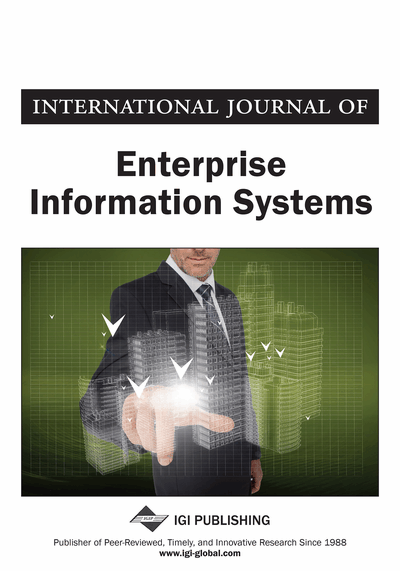A Multi-Attribute Decision-Making Model Using Interval-Valued Intuitionistic Fuzzy Numbers and Attribute Correlation

Sha Fu (School of Information Technology and Management, Hunan University of Finance and Economics, Changsha, China), Xi-long Qu (School of Information Technology and Management, Hunan University of Finance and Economics, Changsha, China), Hang-jun Zhou (School of Information Technology and Management, Hunan University of Finance and Economics, Changsha, China) and Guo-bing Fan (School of Information Technology and Management, Hunan University of Finance and Economics, Changsha, China)
DOI: 10.4018/IJEIS.2018010102
Available
\$37.50
No Current Special Offers

Abstract

This article describes a decision analysis method with attribute correlation and attribute values of interval-valued intuitionistic fuzzy numbers (IVIFN). This considers the correlation between attributes and undetermined attribute weight information was put forward against the case where solution attribute values are interval-valued intuitionistic fuzzy numbers. To achieve a comprehensive consideration of the undetermined evaluation attribute weight information, experts' positive and negative ideal solutions against each attribute are taken as reference points, attribute weight is obtained via decision making trial and evaluation laboratory (DEMATEL) method according to the direct relation matrix provided by experts against attribute sets. On this basis, evaluation information is aggregated by using weighted arithmetic average operators. Then, the closeness degree formula of interval-valued intuitionistic fuzzy numbers relative to the maximum IVIFN is provided by combining the Euclidean distance of IVIFN, thus determining closeness degree and making an ordering of all solutions. Finally, the feasibility and validity of the method proposed in this study are verified via a case analysis of the best information system decision-making.
Article Preview
Top

1. Introduction

The essence of multi-attribute decision-making is to order and pick over a group of (limited) alternatives via a certain way by using the existing decision-making information. In social, economic, technological, military, and other decision-making problems, decision makers often need to provide their preference information of decision-making solutions. However, due to the complexity of actual decision-making environment, as well as the knowledge structure and professional level of decision makers, time and other factors, decision makers usually fail to provide accurate preference information regarding decision-making schemes, there is still a certain degree of hesitation, in this case it would be more suitable to adopt intuitionistic fuzzy numbers or interval-valued intuitionistic fuzzy numbers (IVIFN) to express the decision makers’ preference information.

In recent years, interval-valued intuitionistic fuzzy set-related research has attracted attention of experts and scholars, and has been widely applied in the study of multi-attribute decision-making issues. For instance: Nayagam et al. (2011) introduced and studied a new method for ranking interval-valued intuitionistic fuzzy sets. This method is illustrated by numerical examples and compared with other methods. Park et al. (2011) extend the technique for order preference by similarity to ideal solution (TOPSIS) method to solve multiple attribute group decision making (MAGDM) problems in interval-valued intuitionistic fuzzy environment in which all the preference information provided by the decision-makers is presented as interval-valued intuitionistic fuzzy decision matrices. Ye (2010) proposed a multi-criteria decision-making method based on the weighted correlation coefficients of entropy under an intuitionistic fuzzy environment, as well as providing the evaluation formula of weighted correlation coefficients of the ideal solution, but there will be some difficulties in applying the method to an interval-valued intuitionistic fuzzy environment. Zhang et al. (2012) proposed a decision-making method that is based on interval-valued intuitionistic fuzzy entropy against decision-making problems with completely unknown attribute weight. Gao et al. (2014) proposed an interval-valued intuitionistic fuzzy multi-criteria decision-making method that is based on prospect theory against multi-criteria decision-making problems with completely unknown or partly known criteria weight. Park et al. (2009) and Xu (2010) proposed several decision-making methods based on the correlation coefficient and distance measure of interval-valued intuitionistic fuzzy sets (IVIFSs).

Tomas and Zeng (2013) proposed an extension of the multiplication multi-objective optimization ratio analysis (MULTIMOORA) method based upon interval-valued fuzzy numbers, provided the means for multi-criteria decision making related to uncertain assessments. Chen et al. (2011) developed an approach to tackle multiple criteria group decision-making problems in the context of interval-valued intuitionistic fuzzy sets. Li (2010) developed a nonlinear programming methodology that is based on the technique for order preference by similarity to ideal solution to solve multi-attribute decision-making problems with both ratings of alternatives on attributes and weights of attributes expressed with IVIFSs. Liu and Ren (2015) proposed a new type of multi-attribute decision-making method for intuitionistic fuzzy entropy against the existing intuitionistic fuzzy entropy, which only considers the deviation between membership degree and non-membership degree without considering intuitionistic fuzzy set’s lack of hesitancy degree information. Wang (2006) and Wei (2008) set up an objective programming model based on distance measure and deviation maximization, respectively, and proposed a multi-attribute decision-making method with incomplete attribute weight information and attribute values of interval-valued intuitionistic fuzzy numbers. In the frame of intuitionistic fuzzy set and interval-valued intuitionistic fuzzy sets, Xu (2008), Wang et al. (2009), Nayagam et al. (2011), Li et al. (2014) and Liu et al. (2015) discussed the ordering of intuitionistic fuzzy sets and IVIFSs, and provided the application of these ordering methods in multi-attribute decision-making.

Complete Article List

Search this Journal:
Reset
Volume 18: 4 Issues (2022): Forthcoming, Available for Pre-Order
Volume 17: 4 Issues (2021)
Volume 16: 4 Issues (2020)
Volume 15: 4 Issues (2019)
Volume 14: 4 Issues (2018)
Volume 13: 4 Issues (2017)
Volume 12: 4 Issues (2016)
Volume 11: 4 Issues (2015)
Volume 10: 4 Issues (2014)
Volume 9: 4 Issues (2013)
Volume 8: 4 Issues (2012)
Volume 7: 4 Issues (2011)
Volume 6: 4 Issues (2010)
Volume 5: 4 Issues (2009)
Volume 4: 4 Issues (2008)
Volume 3: 4 Issues (2007)
Volume 2: 4 Issues (2006)
Volume 1: 4 Issues (2005)
View Complete Journal Contents Listing